## Control level sets

### Quick description

From Fubini's theorem one has the identity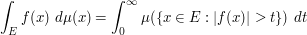(1)

for non-negative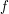. Thus, to estimate an integral, one way to do this is to control the measure of the level sets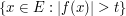for different values of.

A slight variant: the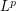norm of a functioncan be expressed by the formula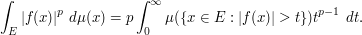(2)

As another variant, one can view (1) as a means to decompose any non-negative functionas a superposition of indicator functions: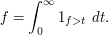Measure theory

### Example 1

Let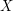be a finite set, and let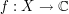be a function. Show that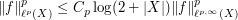for any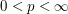.

Solution: we may as well normalize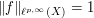, thus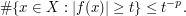If we insert this bound directly into (2) (using counting measure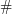instead of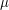) we obtain a logarithmic divergence. But we can improve the bound in two ways. Firstly, when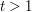, then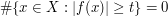, since there is no other non-negative integer less than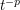. Secondly, we also have the trivial upper bound of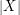, which is superior when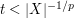. If we then use "divide and conquer" and partition the integral on the right-hand side of (2) into the regions,, and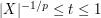, one obtains the claim.

### Example 2

Show that if a functionlies in the weakspaces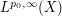and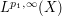for some measure space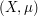and some exponents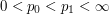, then it lies in the strongspaces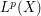for all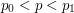.

We need to use "divide and conquer" efficiently in order to 'interpolate' the information we have at the endpoint weakspaces. The idea is that when the functionis large, say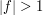, then thenorms increase when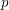increases which is the same as saying that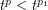whenever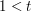and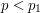. This indicates that we should split the integral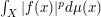into two parts; one integral over the set whereis large and one over its complement. Then the integral over the region whereis large is controlled by the weak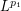norm ofand the integral whereis small is controlled by the weak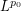norm of.

This can be done in a very elegant fashion by using the description (2) of thenorm of a function. Indeed we can write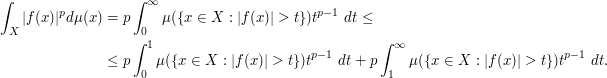For the first term (which corresponds to the set whereis small) we use the weakestimate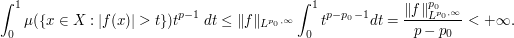Similarly we get for the second term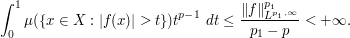Thus we have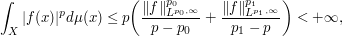and in particular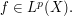More efficiently, one could split the integral at some point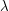instead of the pointand then optimize in the parameter.

### General discussion

This method is closely related to double counting and "interchange integrals or sums".

The method also combines well with dyadic decomposition. Indeed, one easily verifies that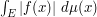is comparable to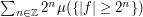, and more generally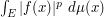is comparable (up to constants depending on) to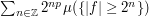.

The Marcinkiewicz interpolation theorem relies heavily on these sorts of level set decompositions.